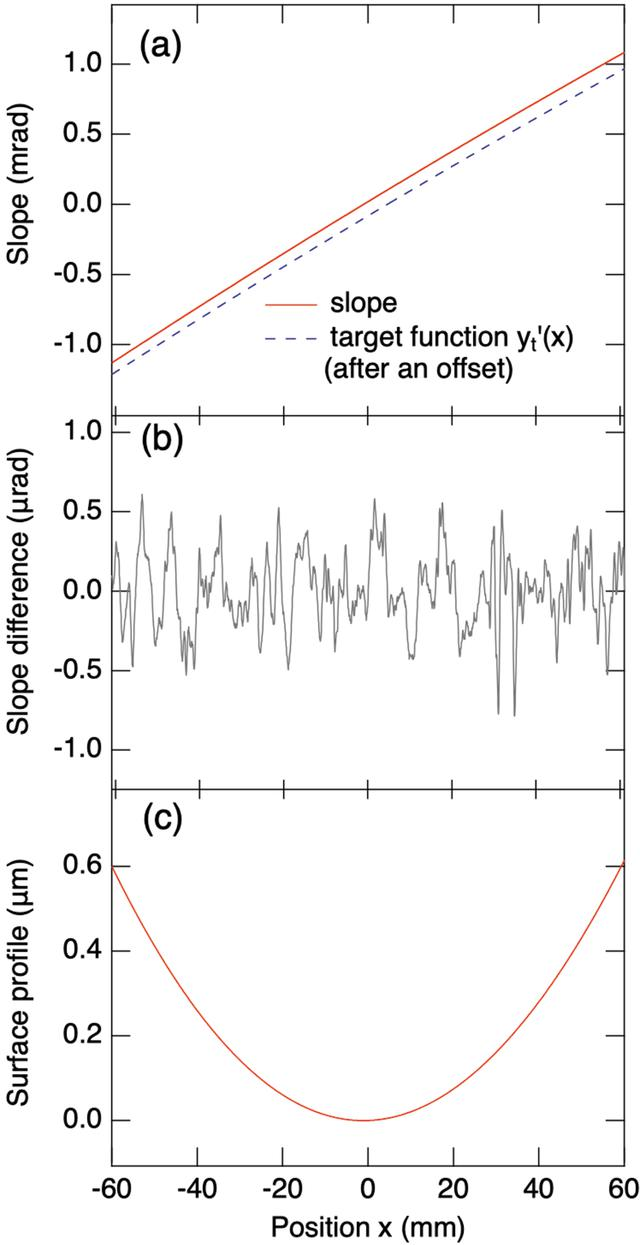disable zoom     view article Figure 7 Slope measurements of an active grating with an in-position LTP. (a) The measured grating slope and its target polynomial slope function yt′(x) = c1x + 2c2x + 3c3x2 + 4c4x3, in which c1 = 1.95 × 10−5, c2 = 9.08 × 10−6, c3 = −3.85 × 10−9 and c4 = 5.94 × 10−14. The function yt′(x) is vertically offset for clarity. (b) The difference between the measured and target slope functions. The r.m.s. of this difference is 0.23 µrad. (c) The profile function of the grating surface obtained from the integration of the measured slope, with the height at the grating centre defined as zero.JOURNAL OFSYNCHROTRONRADIATION
ISSN: 1600-5775
Volume 28| Part 3| May 2021| Pages 977-986